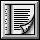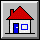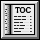5.13 Class STRING`indexing`
```description: "Sequences of characters, accessible through %
%integer indices in a contiguous range."```
`class interface`
`STRING`
`creation `
```frozen make (n: INTEGER)
-- Allocate space for at least n characters.
require
non_negative_size: n >= 0
ensure
empty_string: count = 0```
```make_from_string (s: STRING)
-- Initialize from the characters of s.
-- (Useful in proper descendants of class STRING,
-- to initialize a string-like object from a manifest string.)
require
string_exists: s /= Void```
`feature -- Initialization`
```from_c (c_string: POINTER)
-- Reset contents of string from contents of c_string,
-- a string created by some external C function.
require
C_string_exists: c_string /= Void```
```frozen remake (n: INTEGER)
-- Allocate space for at least n characters.
require
non_negative_size: n >= 0
ensure
empty_string: count = 0```
```make_from_string (s: STRING)
-- Initialize from the characters of s.
-- (Useful in proper descendants of class STRING,
-- to initialize a string-like object from a manifest string.)
require
string_exists: s /= Void```
`feature -- Access`
```hash_code: INTEGER
-- Hash code value
-- (From HASHABLE.)
ensure
good_hash_value: Result >= 0```
```index_of (c: CHARACTER; start: INTEGER): INTEGER
-- Position of first occurrence of c at or after start;
-- 0 if none.
require
start_large_enough: start >= 1;
start_small_enough: start <= count
ensure
non_negative_result: Result >= 0;
at_this_position: Result > 0 implies item (Result) = c;
-- none_before: For every i in start..Result, item (i) /= c
-- zero_iff_absent:
--     (Result = 0) = For every i in 1..count, item (i) /= c```
```item (i: INTEGER): CHARACTER
-- Character at position i
require
good_key: valid_index (i)```
```substring_index (other: STRING; start: INTEGER) : INTEGER
-- Position of first occurrence of other at or after start;
-- 0 if none.```
```infix "@" (i: INTEGER): CHARACTER
-- Character at position i
require
good_key: valid_index (i)```
`feature -- Measurement`
```count: INTEGER
-- Actual number of characters making up the string```
```occurrences (c: CHARACTER): INTEGER
-- Number of times c appears in the string
ensure
non_negative_occurrences: Result >= 0```
`feature -- Comparison`
```is_equal (other: like Current): BOOLEAN
-- Is string made of same character sequence as other?
-- (Redefined from GENERAL.)
require
other_exists: other /= Void```
```infix "<" (other: STRING): BOOLEAN
-- Is string lexicographically lower than other?
-- (False if other is void)
-- (From COMPARABLE.)
require
other_exists: other /= Void
ensure
asymmetric: Result implies not (other < Current)```
```infix "<=" (other: like Current): BOOLEAN
-- Is current object less than or equal to other?
-- (From COMPARABLE.)
require
other_exists: other /= Void
ensure
definition: Result = (Current < other) or is_equal (other);```
```infix ">=" (other: like Current): BOOLEAN
-- Is current object greater than or equal to other?
-- (From COMPARABLE.)
require
other_exists: other /= Void
ensure
definition: Result = (other <= Current)```
```infix ">" (other: like Current): BOOLEAN
-- Is current object greater than other?
-- (From COMPARABLE.)
require
other_exists: other /= Void
ensure
definition: Result = (other < Current)```
```max (other: like Current): like Current)
-- The greater of current object and other
-- (From COMPARABLE.)
require
other_exists: other /= Void
ensure
current_if_not_smaller: (Current >= other) implies (Result = Current)
other_if_smaller: (Current < other) implies (Result = other)```
```min (other: like Current): like Current)
-- The smaller of current object and other
-- (From COMPARABLE.)
require
other_exists: other /= Void
ensure
current_if_not_greater: (Current <= other) implies (Result = Current)
other_if_greater: (Current > other) implies (Result = other)```
```three_way_comparison (other: like Current): INTEGER)
-- If current object equal to other, 0; if smaller,
-- -1; if greater, 1.
-- (From COMPARABLE.)
require
other_exists: other /= Void
ensure
equal_zero: (Result = 0) = is_equal (other);
smaller: (Result = -1) = Current < other;
greater_positive: (Result = 1) = Current > other```
`feature -- Status report`
```empty: BOOLEAN
-- Is string empty?```
```valid_index (i: INTEGER): BOOLEAN
-- Is i within the bounds of the string?```
`feature -- Element change`
```append_boolean (b: BOOLEAN)
-- Append the string representation of b at end.```
```append_character (c: CHARACTER)
-- Append c at end.
ensure
item_inserted: item (count) = c
one_more_occurrence: occurrences (c) = old (occurrences (c)) + 1
item_inserted: has (c)```
```append_double (d: DOUBLE)
-- Append the string representation of d at end.```
```append_integer (i: INTEGER)
-- Append the string representation of i at end.```
```append_real (r: REAL)
-- Append the string representation of r at end.```
```append_string (s: STRING)
-- Append a copy of s, if not void, at end.
ensure
new_count: count = old count + s.count
-- appended: For every i in 1.. s.count,
--     item (old count + i) = s.item (i)```
```fill (c: CHARACTER)
-- Replace every character with c.
ensure
-- allblank: For every i in 1..count, item (i) = Blank```
```head (n: INTEGER)
-- Remove all characters except for the first n;
-- do nothing if n >= count.
require
non_negative_argument: n >= 0
ensure
new_count: count = n.min (old count)
-- first_kept: For every i in 1..n, item (i) = old item (i)```
```insert (s: like Current; i: INTEGER)
-- Add s to the left of position i.
require
string_exists: s /= Void;
index_small_enough: i <= count;
index_large_enough: i > 0
ensure
new_count: count = old count + s.count```
```insert_character (c: CHARACTER; i: INTEGER)
-- Add c to the left of position i.
ensure
new_count: count = old count + 1```
```left_adjust
ensure
new_count: (count /= 0) implies (item (1) /= ' ')```
```put (c: CHARACTER; i: INTEGER)
-- Replace character at position i by c.
require
good_key: valid_index (i)
ensure
insertion_done: item (i) = c```
```put_substring (s: like Current; start_pos, end_pos: INTEGER)
-- Copy the characters of s to positions
-- start_pos .. end_pos.
require
string_exists: s /= Void;
index_small_enough: end_pos <= count;
order_respected: start_pos <= end_pos;
index_large_enough: start_pos > 0
ensure
new_count: count = old count + s.count - end_pos + start_pos - 1```
```right_adjust
-- Remove trailing white space.
ensure
new_count: (count /= 0) implies (item (count) /= ' ')```
```tail (n: INTEGER)
-- Remove all characters except for the last n;
-- do nothing if n >= count.
require
non_negative_argument: n >= 0
ensure
new_count: count = n.min (old count)```
`feature -- Removal`
```remove (i: INTEGER)
-- Remove i-th character.
require
index_small_enough: i <= count;
index_large_enough: i > 0
ensure
new_count: count = old count - 1```
```wipe_out
-- Remove all characters.
ensure
empty_string: count = 0;
wiped_out: empty```
`feature -- Resizing`
```resize (newsize: INTEGER)
-- Rearrange string so that it can accommodate
-- at least newsize characters.
-- Do not lose any previously entered character.
require
new_size_non_negative: newsize >= 0```
`feature -- Conversion`
```to_boolean: BOOLEAN
-- Boolean value;
-- "true" yields true, "false" yields false
-- (case-insensitive)```
```to_double: DOUBLE
-- "Double" value; for example, when applied to "123.0",
-- will yield 123.0 (double)```
```to_integer: INTEGER
-- Integer value;
-- for example, when applied to "123", will yield 123```
```to_lower
-- Convert to lower case.```
```to_real: REAL
-- Real value;
-- for example, when applied to "123.0", will yield 123.0```
```to_upper
-- Convert to upper case.```
`feature -- Duplication`
```copy (other: like Current)
-- Reinitialize by copying the characters of other.
-- (This is also used by clone.)
-- (From GENERAL.)
ensure
new_result_count: count = other.count
-- same_characters: For every i in 1..count,
--     item (i) = other.item (i)```
```substring (n1, n2: INTEGER): like Current
-- Copy of substring containing all characters at indices
-- between n1 and n2
require
meaningful_origin: 1 <= n1;
meaningful_interval: n1 <= n2;
meaningful_end: n2 <= count
ensure
new_result_count: Result.count = n2 - n1 + 1
-- original_characters: For every i in 1..n2-n1,
--     Result.item (i) = item (n1 + i -1)```
`feature -- Output`
```out: STRING
-- Printable representation
-- (From GENERAL.)
ensure
result_not_void: Result /= Void```
`invariant`
```irreflexive_comparison: not (Current < Current);
empty_definition: empty = (count = 0);
non_negative_count: count >= 0```
`end`

 Copyright © 1995, Nonprofit International Consortium for Eiffel mailto:nice@atlanta.twr.com Last Updated: 26 October 1997